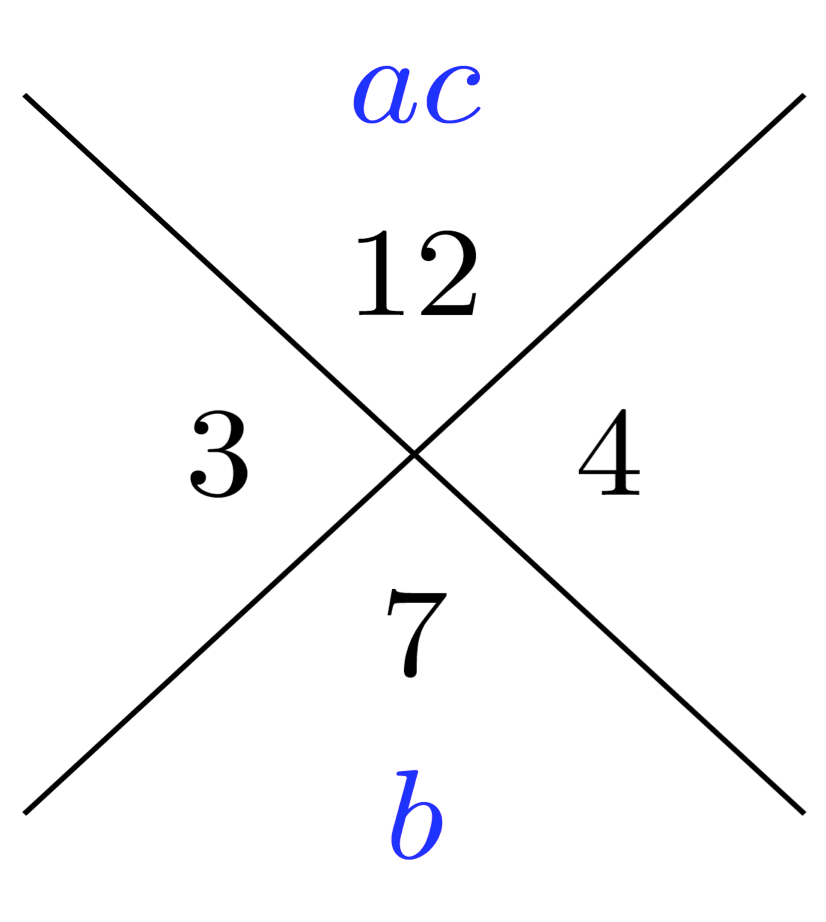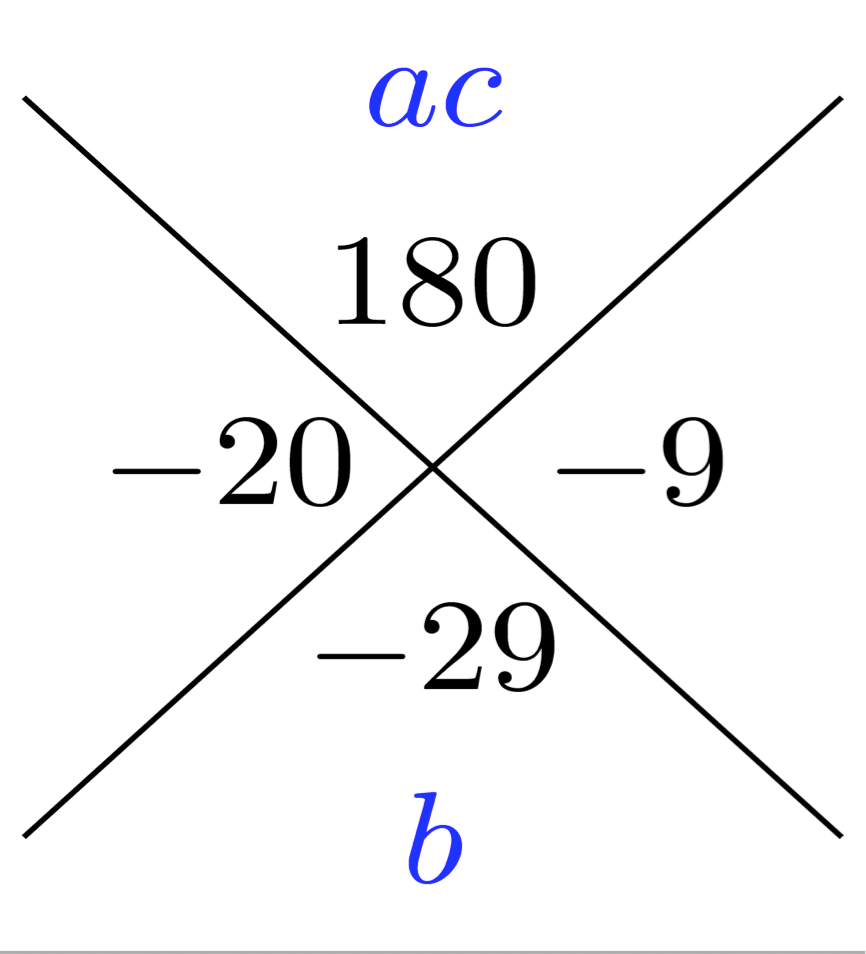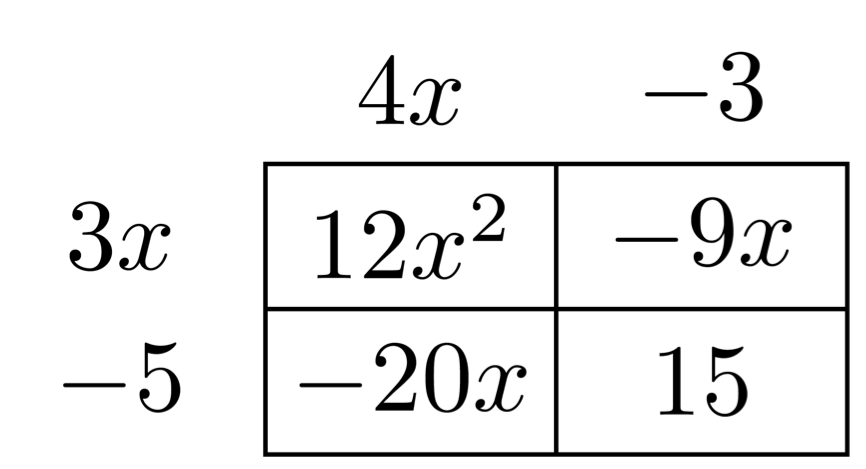# Ways to Factor Trinomials

### Grouping

This quadratic can be factored by grouping. Remembering that the standard from of a trinomial expression is $$ax^2+bx+c$$ . This will become useful as you work through these strategies.

$x^2+7x+12$

First, rewrite this expression with four terms by separating the middle term into two terms with at least one of the two coefficients dividing  evenly into the last term (12). This may require more than one attempt.

$x^2+3x+4x+12$

From there find the GCF of the first two terms and then the GCF of the second two terms. If the terms in the parentheses are the same, then the distribution yields an expression that can be represented as the product of two binomials:

$x(x+3)+4(x+3)=(x+4)(x+3)$

Another way to factor $$x^2+7x+12$$ is a more traditional approach. You are looking for factors of the last term that add up to the coefficient of the 2nd term.  In the diagram below, the number at the top is the product of a and c. The number at the bottom is b. The other two numbers are those that multiply to make 12 and add to 7. Note that the 3 and 4 are the same numbers the seven was split into when the the trinomial was factored by grouping.The numbers 3 and 4 are the constant terms in each  two linear binomials.

Again, the two factors are $$(x+4)(x+3)$$.

### Coefficient of the 1st term is not 1

Here is a second example. This time, the leading coefficient is greater than 1. The steps are a little lengthier.

$12x^2-29x+15$

##### Grouping

Rewrite into four terms. Three and five both divide 15. If 5 is subtracted from 29, the difference is 24, which is factorable by 12.

$12x^2-24x-5x+15=12x(x-2)-5(x-3)$

This did not yield two of the same binomial. This time the 29 is split into 20 and 9. Three divides 9 and 12 and 5 divides 20 and 15.

$12x^2-9x-20x+15=3x(4x-3)-5(4x-3)$

This time there are two binomials that are the same.
This expression is equivalent to $$(3x-5)(4x-3)$$

Using the large X, this procedure begins just like it did for the first example, where the leading coefficient was 1.

The product of the first term, a, and the last term, c is 180, so 180 is placed at the top of the X. The middle term, b, is placed at the bottom.Two numbers whose product is 180 and sum is -29 are -20 and -9.

##### Generic Rectangle

Unlike the first example, this procedure is not complete. A generic rectangle is needed to find the factors. The first term of the trinomial is placed on the top left, the last term is placed on the bottom right. The two numbers from either side of the X are placed in the remaining boxes. Order for those two numbers is not important.Once again, GCFs are needed.  Find the GCF of each column and row. Then check to be sure the products of each row and column are correct when the outside terms are multiplied.   Example: $$4x\cdot 3x = 12x^2$$ Look back to the grouping method and note the similarities.

Once again, the factored form of $$12x^2-29x+15$$ is $$(4x-3)(3x-5)$$